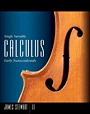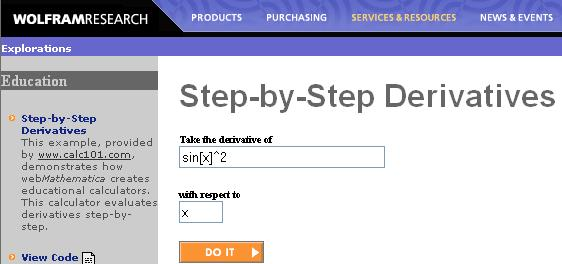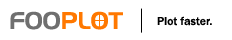ResourcesCourse file for MATH 101 (old exams, ....)Textbook webpageStep-by-Step Derivatives This example, provided by www.calc101.com, demonstrates how webMathematica creates educational calculators. This calculator evaluates derivatives step-by-step.2-D plotting Here you can plot functions, functions in polar coordinates, and parametric equations.